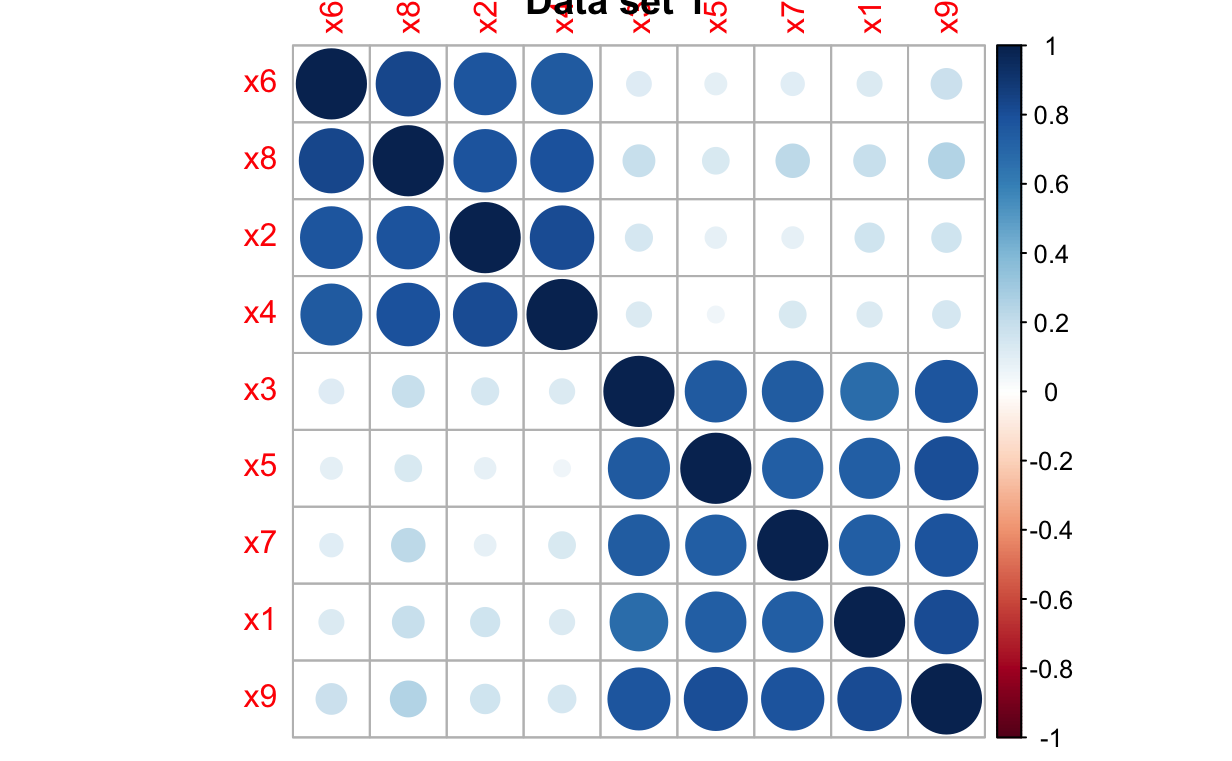In the R notebook illustrating challenges of multiple regression I illustrated why we might want to reduce the number of covariates in our regression. In this notebook I’m going to illustrate a couple of different approaches:

1. Retaining only the covariates that have a “real” relationship with the response variable.
2. Selecting covariates from (relatively) uncorrelated clusters of covariates

## Retaining covariates with a “real” relationship

In the example we’ve been playing with so far, we know that only `x1`, `x2`, and `x3` have a “real” relationship with `y`, because the process we used to generate the data has 0s for the coefficient relating `x4`-`x9` to `y`. Let’s regenerate the data from our last example, with \(R^2 \approx 0.4\), restrict our analysis to only `x1`, `x2`, and `x3` and see if we encounter the instability in parameter estimates and predictions we saw last time.

``````library(tidyverse)
library(reshape2)
library(ggplot2)
library(cowplot)
library(mvtnorm)
library(corrplot)``````
``````## intetcept
##
beta0 <- 1.0
## regression coefficients
##
beta <- c(1.0, -1.0, 1.0, 0.0, 0.0, 0.0, 0.0, 0.0, 0.0)
## pattern of correlation matrix, all non-zero entries are set to saem
## correlation, covariance matrix caldulated from individual variances and a
## single association parameter governing the non-zero correlation coefficients
##
## Note: Not just any pattern will work here. The correlation matrix and
## covariance matrix generated from this pattern must be positive definite.
## If you change this pattern, you may get an error when you try to generate
## data with a non-zero association parameter.
##
Rho <- matrix(nrow = 9, ncol = , byrow = TRUE,
data = c(1,0,1,0,1,0,1,0,1,
0,1,0,1,0,1,0,1,0,
1,0,1,0,1,0,1,0,1,
0,1,0,1,0,1,0,1,0,
1,0,1,0,1,0,1,0,1,
0,1,0,1,0,1,0,1,0,
1,0,1,0,1,0,1,0,1,
0,1,0,1,0,1,0,1,0,
1,0,1,0,1,0,1,0,1
))
## vector of standard deviations for covariates
##
sigma <- rep(1, 9)

## construct a covariance matrix from the pattern, standard deviations, and
## one parameter in [-1,1] that governs the magnitude of non-zero correlation
## coefficients
##
## Rho - the pattern of associations
## sigma - the vector of standard deviations
## rho - the association parameter
##
construct_Sigma <- function(Rho, sigma, rho) {
## get the correlation matris
##
Rho <- Rho*rho
for (i in 1:ncol(Rho)) {
Rho[i,i] <- 1.0
}
## notice the use of matrix multiplication
##
Sigma <- diag(sigma) %*% Rho %*% diag(sigma)
return(Sigma)
}

## set the random number seed manually so that every run of the code will
## produce the same numbers
##
set.seed(1234)

n_samp <- 100
cov_str <- rmvnorm(n_samp,
mean = rep(0, nrow(Rho)),
sigma = construct_Sigma(Rho, sigma, 0.8))

resid <- rep(2.0, n_samp)

y_str <- rnorm(nrow(cov_str), mean = beta0 + cov_str %*% beta, sd = resid)
dat_1 <- data.frame(y_str, cov_str, rep("Strong", length(y_str)))

cov_str <- rmvnorm(n_samp,
mean = rep(0, nrow(Rho)),
sigma = construct_Sigma(Rho, sigma, 0.8))
y_str <- rnorm(nrow(cov_str), mean = beta0 + cov_str %*% beta, sd = resid)
dat_2 <- data.frame(y_str, cov_str, rep("Strong", length(y_str)))

column_names <- c("y", paste("x", seq(1, length(beta)), sep = ""), "Scenario")
colnames(dat_1) <- column_names
colnames(dat_2) <- column_names``````

Now that the data are generated, let’s run the analyses

``````lm_for_pred_1 <- lm(y ~ x1 + x2 + x3, data = dat_1)
lm_for_pred_2 <- lm(y ~ x1 + x2 + x3, data = dat_2)
summary(lm_for_pred_1)``````
``````
Call:
lm(formula = y ~ x1 + x2 + x3, data = dat_1)

Residuals:
Min      1Q  Median      3Q     Max
-5.5057 -1.3596  0.0372  1.0451  4.9972

Coefficients:
Estimate Std. Error t value Pr(>|t|)
(Intercept)   0.8951     0.2076   4.311 3.93e-05 ***
x1            0.9918     0.3274   3.029 0.003150 **
x2           -0.5504     0.2232  -2.466 0.015419 *
x3            1.0247     0.2942   3.483 0.000748 ***
---
Signif. codes:  0 ‘***’ 0.001 ‘**’ 0.01 ‘*’ 0.05 ‘.’ 0.1 ‘ ’ 1

Residual standard error: 2.07 on 96 degrees of freedom
Multiple R-squared:  0.4006,    Adjusted R-squared:  0.3819
F-statistic: 21.39 on 3 and 96 DF,  p-value: 1.079e-10``````
``summary(lm_for_pred_2)``
``````
Call:
lm(formula = y ~ x1 + x2 + x3, data = dat_2)

Residuals:
Min      1Q  Median      3Q     Max
-4.5624 -1.3991 -0.0468  1.3177  4.1804

Coefficients:
Estimate Std. Error t value Pr(>|t|)
(Intercept)   1.0206     0.2001   5.099 1.71e-06 ***
x1            0.6424     0.3398   1.891 0.061704 .
x2           -1.0993     0.1900  -5.787 8.99e-08 ***
x3            1.1957     0.3199   3.737 0.000316 ***
---
Signif. codes:  0 ‘***’ 0.001 ‘**’ 0.01 ‘*’ 0.05 ‘.’ 0.1 ‘ ’ 1

Residual standard error: 1.93 on 96 degrees of freedom
Multiple R-squared:  0.4848,    Adjusted R-squared:  0.4687
F-statistic: 30.11 on 3 and 96 DF,  p-value: 8.283e-14``````

Well, the first thing we discover is that the parameter estimates are not as stable as we might hope. They’re all in the right direction - `x1` and `x3` have positive coefficients, `x2` has a negative coefficient -, but the magnitude of `x2` is a long way off in the first data set and the magnitude of `x1` is a long way off in the second data set. If we compare predictions, here’s what we get:

``````new_data <- data.frame(x1 = 4.0, x2 = 4.0, x3 = 4.0,
x4 = 4.0, x5 = 4.0, x6 = 4.0,
x7 = 4.0, x8 = 4.0, x9 = 4.0)
pred_from_1 <- predict.lm(lm_for_pred_1, new_data)
pred_from_2 <- predict.lm(lm_for_pred_2, new_data)

cat("Prediction from data set 1: ", pred_from_1, "\n",
"Prediction from data set 2: ", pred_from_2, "\n",
"True answer:                ", beta0 + as.matrix(new_data) %*% beta, "\n", sep = "")``````
``````Prediction from data set 1: 6.7596
Prediction from data set 2: 3.975488
That doesn’t look very good either. This isn’t good news, because we know that `x1`, `x2`, and `x3` have an association with `y` and even though we excluded irrelevant covariates our parameter estimates aren’t very stable and our predictions can be pretty variable. Let’s try another approach.
One thing we could observe from the data even if we didn’t know how it was constructed is how the covariates are associated with one another. Let’s visualize those associations using `corrplot()` on each of the data sets.
``corrplot(cor(dat_1[,2:10]), order = "hclust", title = "Data set 1")````corrplot(cor(dat_2[,2:10]), order = "hclust", title = "Data set 2")``